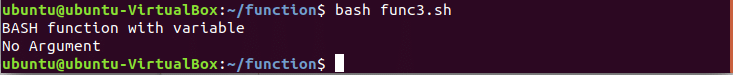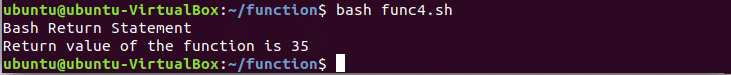BASH Programming

# Use of BASH function that returns a value

Bash Functions can’t return values like other standard programming languages. Bash functions support return statement but it uses different syntax to read the return value.  You can get the value from bash functions in different ways. In this tutorial, you will learn how you can pass string data from bash function to the caller by using different types of bash syntaxes. Open a text editor to test the following bash function examples to understand how string or numeric values can be returned from bash functions.

## Example-1: Using Global Variable

Bash function can return a string value by using a global variable. In the following example, a global variable, ‘retval’ is used. A string value is assigned and printed in this global variable before and after calling the function. The value of the global variable will be changed after calling the function. This is a way of returning string value from a bash function.

function F1()
{
retval='I like programming'
}

retval='I hate programming'
echo \$retval
F1
echo \$retval

Create a bash file named func1.sh with the above code and run the script from the terminal. Here, the output ‘I like programming’ is assigned and printed after function call.## Example-2: Using Function Command

You can receive the return value of a bash function and store it in a variable at the time of calling.  In the following example, a local variable, retval is used and the value of the local variable is return by the function F2 is assigned in a global variable, getval which is printed later.

function F2()
{
local  retval='Using BASH Function'
echo "\$retval"
}

getval=\$(F2)
echo \$getval

Create a bash script named func2.sh with the above code and run the script.## Example-3: Using Variable

In the following example, the return value of the function is set based on the argument variable of the function. Here, a value is passed to the function F3 by using an argument variable, getval1 at the time of function calling. After checking conditional statement, the return value is assigned and printed.

function F3()
{
local arg1=\$1

if [[ \$arg1 != "" ]];
then
retval="BASH function with variable"
else
echo "No Argument"
fi
}

getval1="Bash Function"
F3 \$getval1
echo \$retval
getval2=\$(F3)
echo \$getval2

Create a bash script named func3.sh with the above code and run the script.## Example-4: Using Return Statement

Most of the standard programming language use return statement to return a value from the function. Function values are returned without using any return statement in the above examples. In the following example, return statement is used to return a numeric value from the function F4.  Here, \$? is used to read the value 35 which is returned by the function using return statement.

function F4() {
echo 'Bash Return Statement'
return 35
}

F4
echo "Return value of the function is \$?"

Create a bash script named func4.sh with the above code and run the script.You can use bash functions in various ways to return any string or numeric value after calling the function.